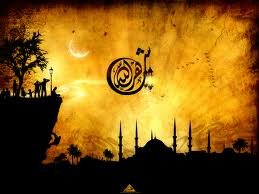Miracles of the Qur'an# THE INITIAL LETTERS “HA-MEEM” AND 19

Seven successive suras begin with the initial letters “Ha-Meem.” These suras are the 40th, 41st, 42, 43rd, 44th, 45th and 46th suras. The verses of all these suras are introduced by two letters: “Ha-Meem

Here, the total number of letters “Ha” and “Meem” used in these 7 suras is 2147 (113×19), and this number is an exact multiple of 19.

The code 19 demonstrates an interlocking mathematical system in the seven suras starting with the initial letters “Ha-Meem.” The system involves both the divisibility of the frequencies of letters by 19, and a unique mathematical formula.

When Milan Sulc added together the values of the digits of all the letters “Ha” and “Meem,” he got the number “113,” which is a multiple of 19. Please see the table below:

 Sura number Ha Meem The total of digits Total 40 64 380 6+4+3+8+0 21 41 48 276 4+8+2+7+6 27 42 53 300 5+3+3+0+0 11 43 44 324 4+4+3+2+4 17 44 16 150 1+6+1+5+0 13 45 31 200 3+1+2+0+0 6 46 36 225 3+6+2+2+5 18 Total 292 1855 113 General Total 2147 (19x113)

If we examine the 42nd sura, we see this sura has a difference. The first verse of this sura is introduced with the initial letters “Ha-Meem,” and the second verse has the initial letters “Ayn-Seen-Qaf” (This sura is the only sura that has initial letters in the second verse). Thus, initial letters “Ha-Meem” are used in the 40th, 41st and 42nd suras in succession, and we can think that the initial letters in the second verse of the 42nd sura (Ayn-Seen-Qaf) divide “Ha-Meem” initialed suras into two groups: three suras (40, 41, 42) and four suras (43, 44, 45, 46) which follow each other. It is very interesting to note that if we divide the suras in this manner, there are 1121 (19×59) letters “Ha Meem” in the suras forming the first group and this number (1121) is an exact multiple of 19, and there are 1026 (19×54) letters “Ha Meem” in the suras forming the second group and this number (1026) is also an exact multiple of 19. Moreover, the total of the digits in the first table below is 59 (When we multiply 59 with the number 19, we get the total of the initials in the first group), and the total of the digits in the second table below is 54 (When we multiply 54 by 19, we get the total of the initials in the second group).

29- It is tablets for people
74-The Hidden, 29

30- Over it is 19
74-The Hidden, 30

The following tables will better demonstrate:

 Sura number Ha Meem The total of the digits Total 40 64 380 6+4+3+8+0 21 41 48 276 4+8+2+7+6 27 42 53 300 5+3+3+0+0 11 Total 165 956 59 General Total 1121 (19 x 59)

 Sura number Ha Meem The total of digits Total 43 44 324 4+4+3+2+4 17 44 16 150 1+6+1+5+0 13 45 31 200 3+1+2+0+0 6 46 36 225 3+6+2+2+5 18 Total 127 899 54 General Total 1026 (19 x 54)